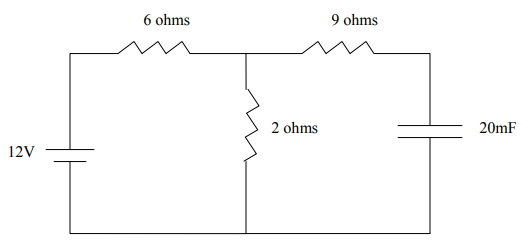## A Capacitor's Voltage

The below diagram is a circuit connected to a 12V voltage source. What is the voltage across the capacitor? Assume steady state.Hint
At steady state, the circuit will act as if the capacitor is an open circuit. Since the capacitor is charged, no more current can flow through it. The 6Ω and 2Ω resistors are in the closed circuit.

Ohm's Law:
$$V=iR$$$At steady state, the circuit will act as if the capacitor is an open circuit. Since the capacitor is charged, no more current can flow through it. The 6Ω and 2Ω resistors are in the closed circuit. $$Re_{q}=R_{1}+R_{2}=6+2=8\Omega$$$
Using Ohm's law, we can solve for the circuit's current:
$$i=\frac{V}{Re_{q}}=\frac{12V}{8\Omega}=1.5A$$$Because no current is flowing through the 9Ω resistor, the voltage across the capacitor is the same across the 2Ω resistor. $$V=iR=1.5A\cdot 2\Omega=3V$$$
$$=3V$$\$